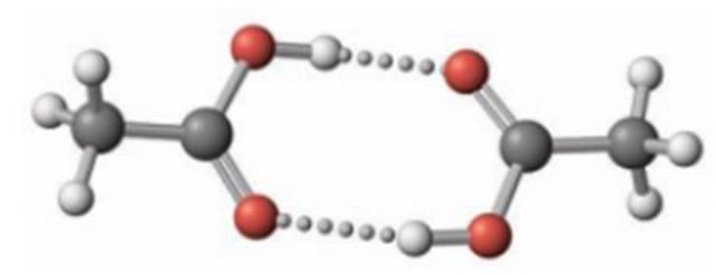Chapter 15, Problem 48GQ

Chapter
Section
Textbook Problem

In the gas phase, acetic acid exists as an equilibrium of monomer and dimer molecules. (The dimer consists of two molecules linked through hydrogen bonds.)The equilibrium constant, Kc, at 25 °C for the monomer-dimer equilibrium2 CH3CO2H ⇄ (CH3CO2H)2has been determined to be 3.2 × 104. Assume that acetic acid is present initially at a concentration of 5.4 × 10−4 mol/L at 25 °C and that no dimer is present initially.(a) What percentage of the acetic acid is converted to dimer?(b) As the temperature increases, in which direction does the equilibrium shift? (Recall that hydrogen-bond formation is an exothermic process.)

(a)

Interpretation Introduction

Interpretation:

The percentage of acetic acid converted to dimer in the given reaction has to be given.

Concept Introduction:

Equilibrium constant in terms of concentrationKC:

aA(g)+bB(g)cC(g)+dD(g)KC=[C]c×[D]d[A]a×[B]b

Le Chatelier’s principle: If equilibrium is disturbed by changing conditions, the system will moves the equilibrium to reverse the change.

Explanation

Given:

2CH3CO2H(CH3CO2H)2KC=3.2×104InitialconcentrationofCH3COOH=5.4×10-4mol/LT=25°C=298K

2CH3CO2H(CH3CO2H)2Initial5.4×10-40Change-2x+xEquilibrium5.4×10-4-2xx

KC=[(CH3CO2H)2][CH3CO2H]23

(b)

Interpretation Introduction

Interpretation:

The direction in which the equilibrium shift as the temperature increases has to be given.

Concept Introduction:

Equilibrium constant in terms of concentrationKC:

aA(g)+bB(g)cC(g)+dD(g)KC=[C]c×[D]d[A]a×[B]b

Le Chatelier’s principle: If equilibrium is disturbed by changing conditions, the system will moves the equilibrium to reverse the change.

Factor’s that effect chemical equilibria:

Concentration – Equilibrium will be affected by changing the concentration of reactant or product. If we increase the concentration of reactant, the system will try to reverse the change by favouring forward reaction and thus increase the concentration of products. Like wise adding products increase yield of reactants.

Temperature – When the temperature increases equilibrium will shift in the endothermic direction, in the direction that absorbs heat. When the temperature decreases equilibrium will shift in the exothermic direction, in the direction that releases heat.

Still sussing out bartleby?

Check out a sample textbook solution.

See a sample solution

The Solution to Your Study Problems

Bartleby provides explanations to thousands of textbook problems written by our experts, many with advanced degrees!

Get Started

Find more solutions based on key concepts